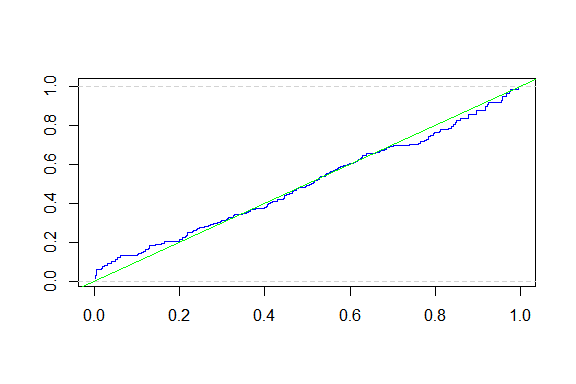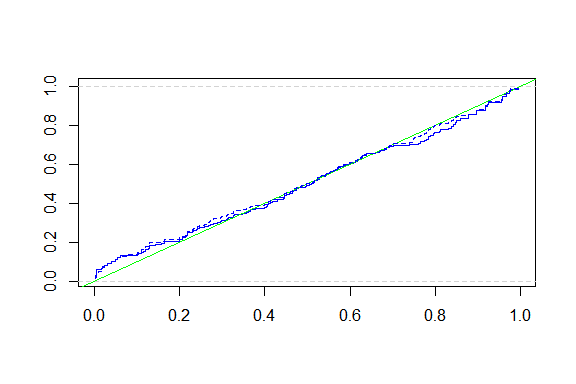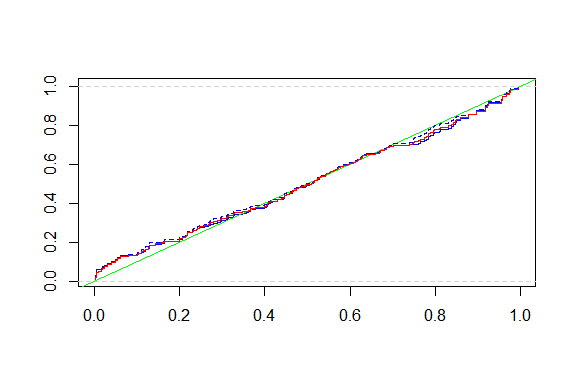Package DTDA.ni

Description [package]

This package implements a non-iterative estimator for the cumulative distribution of a doubly truncated variable, see de Uña-Álvarez (2018). The package is restricted to interval sampling.

de Uña-Álvarez J. (2018) A Non-iterative Estimator for Interval Sampling and Doubly Truncated Data. In: Gil E., Gil E., Gil J., Gil M. (eds) The Mathematics of the Uncertain. Studies in Systems, Decision and Control, vol 142. Springer, Cham, pp. 387-400.

DTDAni(x, u , tau)

Description [function DTDAni]

This function calculates the non-iterative estimator for the cumulative distribution of a doubly truncated variable proposed by de Uña-Álvarez (2018). The package works for interval sampling.

Details [function DTDAni]

The function DTDAni is adapted to the presence of ties. It can be used to compute the direct $$(Fd)$$ and the reverse $$(Fr)$$ estimators; see the example below. Both curves are valid estimators for the cumulative distribution $$(F)$$ of the doubly truncated variable. Weighted estimators $$Fw = w*Fd + (1-w)*Fr$$ with $$0<w<1$$ are valid too, the choice $$w=1/2$$ being recommended in practice (de Uña-Álvarez, 2018).

Usage

In order to use this package, install it and load the library with: library(DTDA.ni)

Parameters

• x: Numeric vector corresponding the variable of ultimate interest.
• u: Numeric vector corresponding to the left truncation variable.
• tau: Sampling interval width. The right truncation values will be internally calculated as v = u + tau.

Return

• x : The distinct values of the variable of interest.
• nx : The absloute frequency of each x value.
• cumprob : The estimated cumulative probability for each x value.
• P : The auxiliary Pi used in the calculation of the estimator.
• L : The auxiliary Li used in the calculation of the estimator.

Usage


library(DTDA.ni)

# Generating data which are doubly truncated:
N <- 250
x0 <- runif(N)           # Original data
u0 <- runif(N, -0.25, 0.5) # Left-truncation times
tau <- 0.75               # Interval width
v0 <- u0 + tau

x <- x0[u0 <= x0 & x0 <= v0]
u <- u0[u0 <= x0 & x0 <= v0]
v <- v0[u0 <= x0 & x0 <= v0]
n <- length(x)  # Final sample size after the interval sampling

# Create an object wit DTDAni function
res <- DTDAni(x, u, tau)
#> Call:
#> DTDAni(x = x, u = u, tau = tau)
plot(res, ecdf = FALSE) # Plot without ecdf (Default)
abline(a = 0, b = 1, col = "green")  # The true cumulative distribution# Calculating the reverse estimator:
plot(res)

res2 <- DTDAni(-x, -u - 0.75, 0.75)
#> Call:
#> DTDAni(x = -x, u = -u - 0.75, tau = 0.75)
abline(a = 0, b = 1, col = "green")
lines(-res2$x, 1 - res2$cumprob, type = "s", col = "blue", lty = 2)# Weigthed estimator (recommended):

w <- 1/2

k <- length(res$x) Fw <- w * res$cumprob + (1 - w) * (1 - res2$cumprob[k:1]) plot(res) abline(a = 0, b = 1, col = "green") lines(-res2$x, 1 - res2$cumprob, type = "s", col = "blue", lty = 2) lines(res$x, Fw, type = "s", col = 2)# Using res$P and res$L to compute the estimator:

k <- length(res$x) F <- rep(1, k) for (i in 2:k) { F[i] <- (F[i - 1] - res$P[i - 1]) / res$L[i - 1] + res$P[i - 1]
}

F0 <- F / max(F)  # This is equal to res\$cumprob

Author(s)

• de Uña Álavarez, Jacobo.
• Soage González, José Carlos.

Maintainer: José Carlos Soage González (jsoage@uvigo.es)

Acknowledgements

• Jacobo de Uña-Álvarez was supported by Grant MTM2014-55966-P, Spanish Ministry of Economy and Competitiveness .

• José Carlos Soage was supported by Red Tecnológica de Matemática Industrial (Red TMATI), Cons. de Cultura, Educación e OU, Xunta de Galicia (ED341D R2016/051) and by Grupos de Referencia Competitiva, Consolidación y Estructuración de Unidades de Investigación Competitivas del SUG, Cons. de Cultura, Educación e OU, Xunta de Galicia (GRC ED431C 2016/040).